Click to Chat

1800-1023-196

+91-120-4616500

CART 0

• 0

MY CART (5)

Use Coupon: CART20 and get 20% off on all online Study Material

ITEM
DETAILS
MRP
DISCOUNT
FINAL PRICE
Total Price: Rs.

There are no items in this cart.
Continue ShoppingFirst Order Reactions

Table of Content

Half-Time or Half-Life Period of a First order Reaction

Some Simple First Order Reactions

Related ResourcesA reaction is said to be first order if its rate is determined by the change of one concentration term only.

One can say that  a first order reaction is one whose rate varies as 1st power of the concentration of the reactant i.e. the rate increases as number of times as the number of times the concentration of reactant is increased.

Consider the reaction

A → products

Let

[A]O   = Initaial Concentration of A

[A]t = The concentration of A after time t

For  the reaction to be of  first order..

...(i)

Intigrating this equation

we get

ln [A] - ln[A]o = -K(t-0)

…...... (ii)

...(iii)

....(iv)

On comparing the equation number (ii) with equation of straight line i.e. y=mx+c we come to know that , If we plot a graph between log [A]o/[A] vs t, the slope of line= k/2.303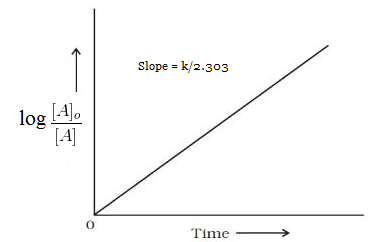Refer to the following video for first order reactions

Unit of rate constant for first order reaction ( k) = min–1 or s-1

The rate constant of a first order reaction has only time unit. It has no concentration unit.

This means the numerical value of k for a first order reaction is independent of the unit in which concentration is expressed.

If concentration unit is changed the numerical value of k for a first order reaction will not change.

However, it would change with  change in time unit. Say, k is 6.0  10–3 min then it may also be written as 110–4 s–1 i.e. numerical value of k will decrease 60 times if time unit is changed from however to minute or from minute to second.

Half-Time or Half-Life Period of a First order ReactionThe half-time of a reaction is defined as the time required to reduce the concentration of the reactant to half of its initial value. It is denoted by the symbol t1/2. Thus,

For first order reaction, we know that

at  half life of reaction,

t = t½ & [A] = [A]o/2

So

Since k is a constant for a given reaction at a given temperature and the expression lacks any concentration term so half-time of a 1st order reaction is a constant independent of initial concentration of reactant.

This means if we start with 4 mole L–1 of a reactant reacting by first-order kinetics and after 20 minute it is reduced to 2 mole L–1 will also be 20 minute.

That is, after 20 minutes from the start of reaction the concentration of the reactant will be 2 mole L–1, after 40 minutes from the start of reaction of concentration is 1 mole L-1. After 60 minutes from the start of reaction the concentration of the reactant will be reduced to 0.5 mole L–1. In other words, if during 20 minute 50% of the reaction completes, then in 40 minute 75%, in 60 minute 85.5% of the reaction and on will complete as shown with following plot.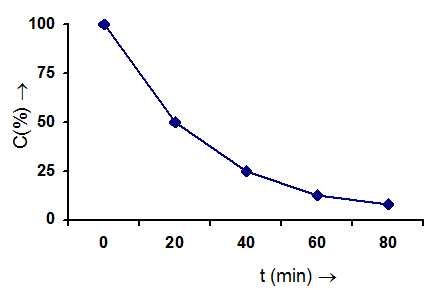Examples of first order reactions

Decomposition of H2O2 in aqueous solution

H2O2  →  H2O + 1/2  O2

Hydrolysis of methyl acetate in presence of mineral acids.

CH3COOCH3 + H2O   →   CH3COOH + CH3OH

Inversion of cane sugar in presence of mineral acids.

C12H22O11 + H2O      C6H12O6 + C6H12O6

Decomposition of ammonium nitrite in aqueous solution.

NH4NO2  →  N2 + 2H2O

Hydrolysis of diazo derivatives.

C5H5N = NCl + H2O  →  C6H5OH + N2 + HCl

Note:    In case of gases, pressure can be used in place of concentration.

Some Simple First Order Reactions

As we have to generally deal with only first order reactions, we examine some of these reactions from the point of calculating the rate constant based on different experimental data.

Now we present several problems in which we shall learn how to calculate the rate constant of reactions based on the  variety of data given.

We are given a first order reaction A →  B+C where we assume that A,B and C are gases. The data given  to us is

Time

0

t

Partial pressure of A

P1

P­2

And we have to find the rate constant of the reaction.

Since A is a gas and assuming it to be ideal, we can state that PA = [A]RT

[From PV = nRT]. \ at t = 0, P1 = [A]o RT and at t = t, P2 = [A]tRT. Thus the ratio of the concentration of A at two different time intervals is equal to the ratio of its partial pressure at those same time intervals.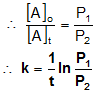Now we consider the same reaction with different set of data A → B +C

Time

0

t

Total pressure  of A +B+C

P1

P2

Find k.

In this we are given total pressure of the system at  these time intervals. The total pressure obviously includes the pressure of A, B and C. At t = 0, the system  would only have A. Therefore the total pressure at t = 0 would be the initial pressure of A \ P1 is the initial pressure of A. At time t let us assume that some moles of A decomposed to give B and C because of which its pressure is reduced by an amount x while that of B and C is increased by x each. That isNow let us move on to the next case. In this case we have A →  B +C

Time

t

Total pressure  of A+B+C

P2

P3

Find k.

Here  means that the  reaction is complete. Now we have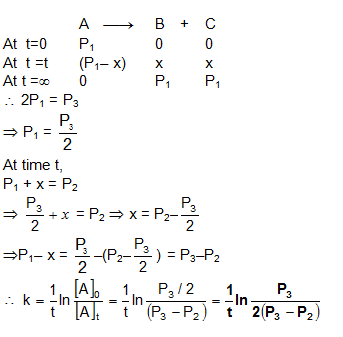Now let us assume that A,B and C are substances present in a solution.

From a solution of A, a certain amount of the solution (small amount) is taken and titrated with a suitable reagent that reacts with A. The volume of the reagent used is V1 at t = 0 and V2 at t = t

Time

0

T

Volume of reagent

V1

V2

The reagent reacts only with A.  Find k.

It can be understood that the volume of the reagent consumed is directly proportional to the concentration of A. Therefore the ratio of volume of the reagent consumed against A at t=0 and t = t is equal to  [A]0 /[A]Now consider this situation A → B+C

Time

T

Volume of reagent

V2

V3

Reagent reacts with all A,B and C and have ‘n’ factors in the ratio of 1:2:3 with the reagent. Find k.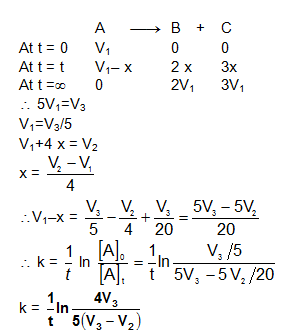Now, we will consider a reaction A B+C which is catalysed by D. We will assume in this problem that the concentration of the catalyst remains constant throughout. The data given to us is

Time

0

t

Volume of reagent

V1

V2

V3

The reagent reacts with all (A,B,C and D). Assume that ‘n’ factor of A,B and C are in the ratio of 1:1:1 and that of D is not known. Find k.

Let VA be the volume of the reagent required by A initially and VD be the volume required
by D.

\V1 = VA + VD

V2 = (VA – x) + x + x +VD (x is the volume of the reagent required for those moles of A that have reacted to  give B and C).

V3 = 2VA+VD

We can see that, V3–V1 = VANow we  shall see how to find the rate constant of a reaction using a very different set of data.

There are some organic compounds which have a property of rotating a plane polarized light in a particular direction by a particular value. The compounds are called optically active compounds. One reaction in which an optically active substance converts to some other optically active substance is,

Sucrose  Glucose + Fructose

Sucrose, Glucose and Fructose are all optically active and while  the first two compounds are dextro rotatory (rotating the plane polarised light in the right hand direction  and the last is laevo rotatory (rotating the plane polarized light in the left hand direction). All the three compounds rotate the plane polarised light by different angles and their rotation is directly proportional to concentration.

Now the problem is S →   G + F and the data is

Time

0

t

Rotation of  sucrose

r0

rt

Find k.

Let the rotation of Sucrose be  r1° per mole and the initial moles of Sucrose be a.

r0 = ar10

Let the moles of Sucrose that is converted to Glucose and Fructose be x.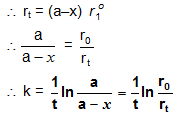Question 1:

Rate of first order reaction.

a. depends on concentration of reactants.

b. depends on concentration of products.

c. depends on concentration of both reactant and product.

d. does not depend on concentrations.

Question 2:

Unit of rate constant for first order reaction is

b. mol2 L-1 time-1.

c. mol L-1 time-1.

d. time-1.

Question 3:

If we plot ln [A]o/[A] vs t, we get a straight line with slope =

a. rate constant

b. arrhenius constant

c. temperature coefficient

d. equilibrium constant

Question 4:

For zero order reaction d[A] =

a. -kdt

b.  -k[A]0

c.-k[A]0dt

d. -k[A]0Q.1

Q.2

Q.3

Q.4

a

d

a

a

Related Resources

Refer to the Books of Chemistry for IIT JEE

To read more, Buy study materials of Chemical Kinetics comprising study notes, revision notes, video lectures, previous year solved questions etc. Also browse for more study materials on Chemistry here.### Course Features

• 731 Video Lectures
• Revision Notes
• Previous Year Papers
• Mind Map
• Study Planner
• NCERT Solutions
• Discussion Forum
• Test paper with Video Solution